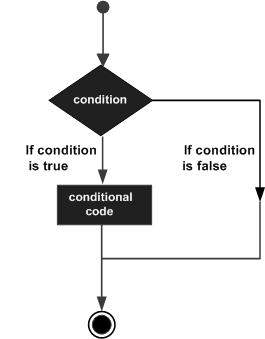# Learn JavaScript for Web Development

## JavaScript If...Else

While writing a program, there may be a situation when you need to adopt one out of a given set of paths. In such cases, you need to use conditional statements that allow your program to make correct decisions and perform right actions.

JavaScript supports conditional statements which are used to perform different actions based on different conditions. Here we will explain the if..else statement.

### Flow Chart of if-else

The following flow chart shows how the if-else statement works.JavaScript supports the following forms of if..else statement −

• if statement

• if...else statement

• if...else if... statement.

### if statement

The if statement is the fundamental control statement that allows JavaScript to make decisions and execute statements conditionally.

Syntax

The syntax for a basic if statement is as follows −

if (expression){
Statement(s) to be executed if expression is true
}

Here a JavaScript expression is evaluated. If the resulting value is true, the given statement(s) are executed. If the expression is false, then no statement would be not executed. Most of the times, you will use comparison operators while making decisions.

See this example:

<html>
<body>

<script type="text/javascript">
<!--
var age = 20;

if( age > 18 ){
document.write("<b>Qualifies for driving</b>");
}
//-->
</script>

<p>Set the variable to different value and then try...</p>
</body>
</html>

Output

Qualifies for driving Set the variable to different value and then try...

### if...else statement:

The 'if...else' statement is the next form of control statement that allows JavaScript to execute statements in a more controlled way.

Syntax

if (expression){
Statement(s) to be executed if expression is true
}

else{
Statement(s) to be executed if expression is false
}

Here JavaScript expression is evaluated. If the resulting value is true, the given statement(s) in the ‘if’ block, are executed. If the expression is false, then the given statement(s) in the else block are executed.

See this example:

Try the following code to learn how to implement an if-else statement in JavaScript.

See this example:

<html>
<body>

<script type="text/javascript">
<!--
var age = 15;

if( age > 18 ){
document.write("<b>Qualifies for driving</b>");
}

else{
document.write("<b>Does not qualify for driving</b>");
}
//-->
</script>

<p>Set the variable to different value and then try...</p>
</body>
</html>

Output:

Does not qualify for driving Set the variable to different value and then try...

### if...else if... statement

The if...else if... statement is an advanced form of if…else that allows JavaScript to make a correct decision out of several conditions.

Syntax

The syntax of an if-else-if statement is as follows −

if (expression 1){
Statement(s) to be executed if expression 1 is true
}

else if (expression 2){
Statement(s) to be executed if expression 2 is true
}

else if (expression 3){
Statement(s) to be executed if expression 3 is true
}

else{
Statement(s) to be executed if no expression is true
}

There is nothing special about this code. It is just a series of if statements, where each if is a part of the else clause of the previous statement. Statement(s) are executed based on the true condition, if none of the conditions is true, then the else block is executed.

See thi9s example:

Try the following code to learn how to implement an if-else-if statement in JavaScript.

See this example:

<html>
<body>

<script type="text/javascript">
<!--
var book = "maths";
if( book == "history" ){
document.write("<b>History Book</b>");
}

else if( book == "maths" ){
document.write("<b>Maths Book</b>");
}

else if( book == "economics" ){
document.write("<b>Economics Book</b>");
}

else{
document.write("<b>Unknown Book</b>");
}
//-->
</script>

<p>Set the variable to different value and then try...</p>
</body>
<html>

Output:

Maths Book Set the variable to different value and then try...